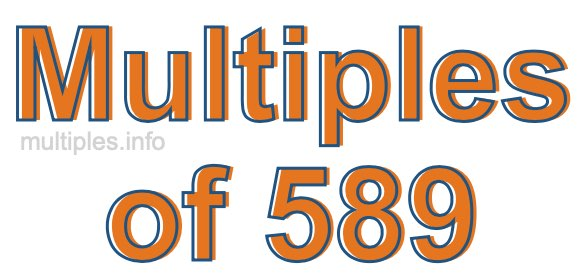Multiples of 589Welcome to the Multiples of 589 page. Here we will first teach you everything you will ever need to know about the multiples of 589, and then give you a study guide summary of everything we taught you to make sure you remember it all. Use this page to look up facts and learn information about the multiples of 589. This page will make you a multiples of five hundred eighty-nine expert!

Definition of Multiples of 589
Multiples of 589 are all the numbers that when divided by 589 equal an integer. Each of the multiples of 589 are called a multiple. A multiple of 589 is created by multiplying 589 by an integer.

Therefore, to create a list of multiples of 589, you start with 1 multiplied by 589, then 2 multiplied by 589, then 3 multiplied by 589, and so on for as long as you want. Thus, the list of the first five multiples of 589 is 589, 1178, 1767, 2356, and 2945. To see a larger list of multiples of 589, see the printable image of Multiples of 589 further down on this page. We also have a category where you can choose any nth multiple of 589.

Multiples of 589 Checker
The Multiples of 589 Checker below checks to see if any number of your choice is a multiple of 589. In other words, it checks to see if there is any number (integer) that when multiplied by 589 will equal your number. To do that, we divide your number by 589. If the the quotient is an integer, then your number is a multiple of 589.

Is  a multiple of 589?

Least Common Multiple of 589 and ...
A Least Common Multiple (LCM) is the lowest multiple that two or more numbers have in common. This is also called the smallest common multiple or lowest common multiple and is useful to know when you are adding our subtracting fractions. Enter one or more numbers below (589 is already entered) to find the LCM.

Check out our LCM Calculator if you need more details about the Least Common Multiple or if you need the LCM for different numbers for adding and subtraction fractions.

nth Multiple of 589
As we stated above, 589 is the first multiple of 589, 1178 is the second multiple of 589, 1767 is the third multiple of 589, and so on. Enter a number below to find the nth multiple of 589.

th multiple of 589

Multiples of 589 vs Factors of 589
589 is a multiple of 589 and a factor of 589, but that is where the similarities end. All postive multiples of 589 are 589 or greater than 589. All positive factors of 589 are 589 or less than 589.

Below is the beginning list of multiples of 589 and the factors of 589 so you can compare:

Multiples of 589: 589, 1178, 1767, 2356, 2945, etc.

Factors of 589: 1, 19, 31, 589

As you can see, the multiples of 589 are all the numbers that you can divide by 589 to get a whole number. The factors of 589, on the other hand, are all the whole numbers that you can multiply by another whole number to get 589.

It's also interesting to note that if a number (x) is a factor of 589, then 589 will also be a multiple of that number (x).

Multiples of 589 vs Divisors of 589
The divisors of 589 are all the integers that 589 can be divided by evenly. Below is a list of the divisors of 589.

Divisors of 589: 1, 19, 31, 589

The interesting thing to note here is that if you take any multiple of 589 and divide it by a divisor of 589, you will see that the quotient is an integer.

Multiples of 589 Table
Below is an image of the first 100 multiples of 589 in a table. The table is in chronological order, column by column. The first column has the first ten multiples of 589, the second column has the next ten multiples of 589, and so on.The Multiples of 589 Table is also referred to as the 589 Times Table or Times Table of 589. You are welcome to print out our table for your studies.

Negative Multiples of 589
Although not often discussed or needed in math, it is worth mentioning that you can make a list of negative multiples of 589 by multiplying 589 by -1, then by -2, then by -3, and so on, to get the following list of negative multiples of 589:

-589, -1178, -1767, -2356, -2945, etc.

Multiples of 589 Summary
Below is a summary of important Multiples of 589 facts that we have discussed on this page. To retain the knowledge on this page, we recommend that you read through the summary and explain to yourself or a study partner why they hold true.

There are an infinite number of multiples of 589.

A multiple of 589 divided by 589 will equal a whole number.

589 divided by a factor of 589 equals a divisor of 589.

The nth multiple of 589 is n times 589.

The largest factor of 589 is equal to the first positive multiple of 589.

589 is a multiple of every factor of 589.

589 is a multiple of 589.

A multiple of 589 divided by a divisor of 589 equals an integer.

589 divided by a divisor of 589 equals a factor of 589.

Any integer times 589 will equal a multiple of 589.

Multiples of a Number
Here you can get the multiples of another number, all with the same attention to detail as we did for multiples of 589 on this page.

Multiples of
Multiples of 590
Did you find our page about multiples of five hundred eighty-nine educational? Do you want more knowledge? Check out the multiples of the next number on our list!